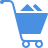Cart (0)

Each independent variable in a regression model has a regression coefficient, which is often denoted by “b.” Each “b” value (regression coefficient) measures the average relationship between the dependent variable and independent variable when all other things are kept constant. If the regression coefficient of media spending is 2, it means, on the average, one unit of change in media spending will cause a change in sales by 2.

Previous Entry
Next Entry